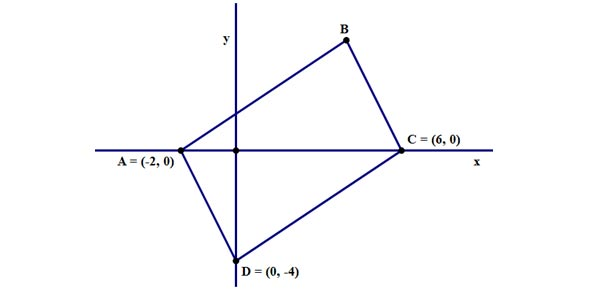# Coordinate Geometry SEC 3 Quiz 2

9 Questions | Total Attempts: 116SettingsWelcome to this Coordinate Geometry Quiz 2! This is a quiz to review your online learning experience. All the Best! Mdm Tham

• 1.
The line y = x –6 meets the curve x^2+y^2-6x+8y=0 at the points A and B. Find the length of AB.    Give your answer in  3 significant figures.
• 2.
The vertices of a triangle are A(-2,3), B(5,7) and C(4,0).  Find  the equation of the line through B parallel to AC                                       Give your answer in the form : y=mx+c with no spaces in between. Inpt the value of m and c as decimal.
• 3.
This question is Part (i) of  a main question. Do note down the information and the answer you have obtained here. ABCD is a parallelogram, labeled anticlockwise such that A and C are the points (-1,5) and (5,1) respectively. Find the coordinates of the midpoint of AC.         Give your answer as Eg '(1,2)' No spaces allowed
• 4.
This question is Part (ii) of  a main question. Do note down the information and the answer you have obtained here. ABCD is a parallelogram, labeled anticlockwise such that A and C are the points (-1,5) and (5,1) respectively.  Given that BD is parallel to the line whose equation is 5x + y – 2 = 0, find  the equation of BD.      Give your answer in the form : y=mx+c with no spaces in between.
• 5.
This question is Part (iii) of  a main question. Do note down the information and the answer you have obtained here. ABCD is a parallelogram, labeled anticlockwise such that A and C are the points (-1,5) and (5,1) respectively.  Given that BD is parallel to the line whose equation is 5x + y – 2 = 0, you have obtained the equation of BD previuosly.    Given that BC is perpendicular to AC, find the equation of BC.         Give your answer in the form : y=mx+c with no spaces in between. Give the value of m and c in decimal.
• 6.
This question is Part (iv) of  a main question. Do note down the information and the answer you have obtained here. ABCD is a parallelogram, labeled anticlockwise such that A and C are the points (-1,5) and (5,1) respectively.  Given that BD is parallel to the line whose equation is 5x + y – 2 = 0, you have obtained the equation of BD and BC previously.    Find the coordinate of B and D. Give your answer as Eg '(1,2)and(3,4)' No spaces allowed.
• 7.
A(2, 6), B(9, 5) and C are points on a circle with diameter AC. Given that          AB = BC, find the coordinates of point(s) C. Give your answer as Eg '(1,2)' No spaces allowed.
• 8.
Write down the coordinates of the centre of the circle x^2+y^2-2x-6y+1=1. Give your answer as Eg '(1,2)' No spaces allowed.
• 9.
Find the equation of the circle whose centre is(-3,4) and touches the y-axis. Give in the form of ax^2+by^2+cx+dy+e=0. No spaces allowed.
Related TopicsBack to top